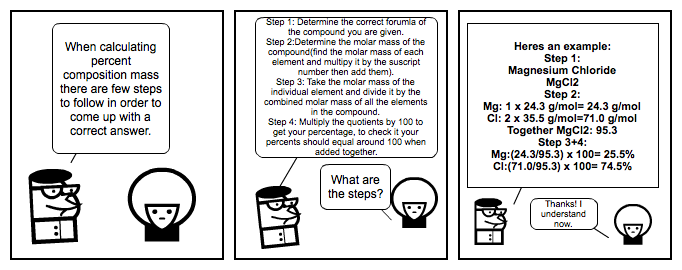## Stripview

0 ThumbsUp

### Chemistry

anonymous on 9. Nov, 2018 — Lang: English• #### Transcript

Expand

double click to write...

When calculating percent composition mass there are few steps to follow in order to come up with a correct answer.

double click to write...

What are the steps?

Step 1: Determine the correct forumla of the compound you are given.
Step 2:Determine the molar mass of the compound(find the molar mass of each element and multipy it by the suscript number then add them).
Step 3: Take the molar mass of the individual element and divide it by the combined molar mass of all the elements in the compound.
Step 4: Multiply the quotients by 100 to get your percentage, to check it your percents should equal around 100 when added together.

double click to write...

Heres an example:
Step 1:
Magnesium Chloride
MgCl2
Step 2:
Mg: 1 x 24.3 g/mol= 24.3 g/mol
Cl: 2 x 35.5 g/mol=71.0 g/mol
Together MgCl2: 95.3
Step 3+4:
Mg:(24.3/95.3) x 100= 25.5%
Cl:(71.0/95.3) x 100= 74.5%

double click to write...

Thanks! I understand now.

Tags:
SG_Tournament